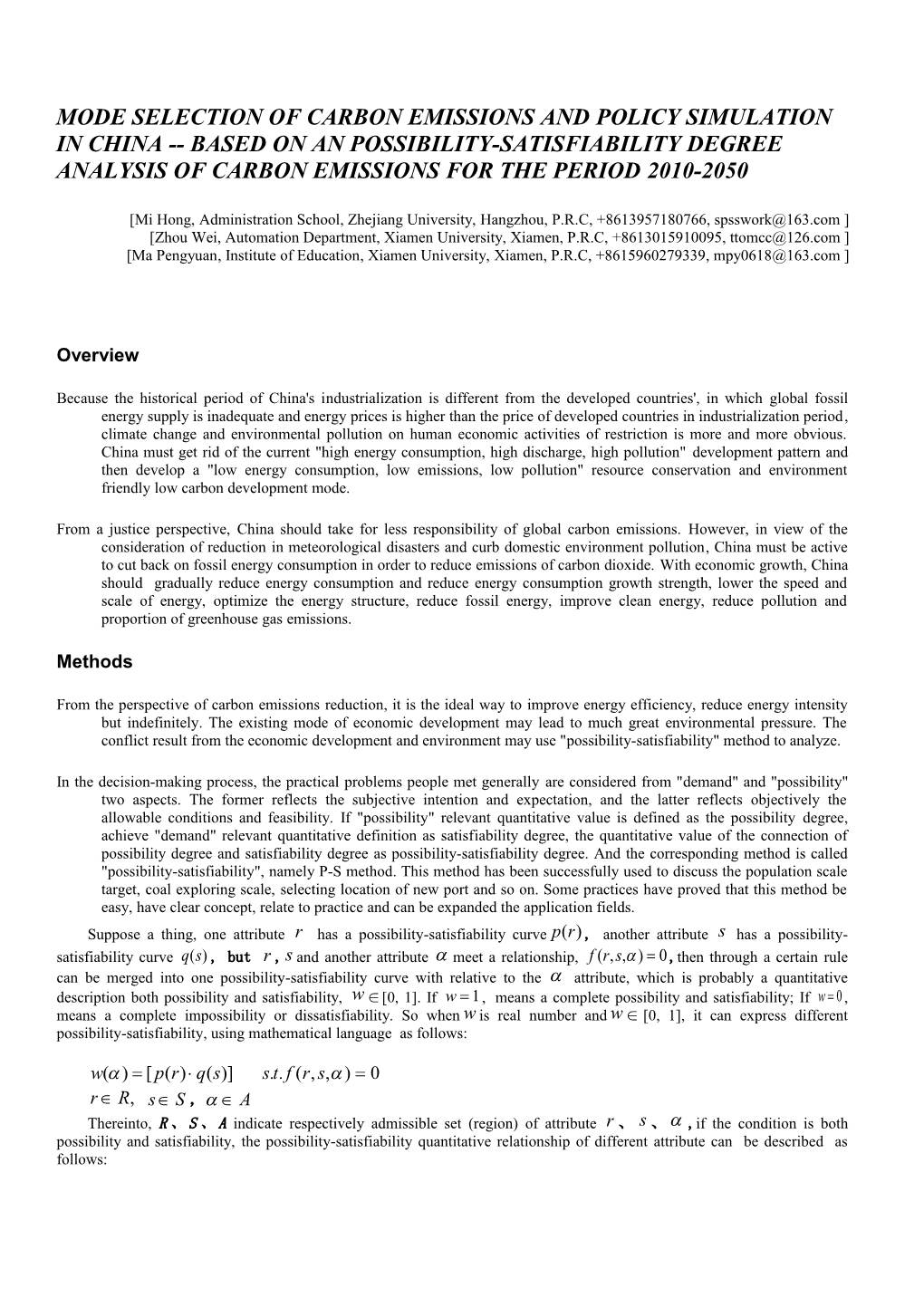# Mode Selection of Carbon Emissions and Policy SIMULATION in China Based on AN1mode selection of carbon emissions and policy SIMULATION IN China ------based on AN possibILITY-satisfIaBILITY degree analysis of carbon emissions for the period 2010-2050

mode selection of carbon emissions and policy SIMULATION IN China --based on AN possibILITY-satisfIaBILITY degree analysis of carbon emissions for the period 2010-2050

[Mi Hong, Administration School, Zhejiang University, Hangzhou, P.R.C, +8613957180766, ]

[Zhou Wei, Automation Department, XiamenUniversity, Xiamen, P.R.C, +8613015910095, ]

[Ma Pengyuan, Institute of Education, XiamenUniversity, Xiamen, P.R.C, +8615960279339, ]

### In the decision-making process, the practical problems people met generally are considered from "demand" and "possibility" two aspects.The former reflects the subjective intention and expectation, and the latter reflects objectively the allowable conditions and feasibility.If "possibility" relevant quantitative value is defined as the possibility degree,achieve "demand" relevant quantitative definition as satisfiability degree, the quantitative value of the connection of possibility degree and satisfiability degree as possibility-satisfiability degree.And the corresponding method is called "possibility-satisfiability", namely P-S method. This method has been successfully used to discuss the population scale target,coal exploring scale, selecting location of newport and so on. Some practices have proved that this method be easy, have clear concept, relate to practice and can be expanded the application fields.

Suppose a thing, one attribute has a possibility-satisfiability curve, another attribute has a possibility-satisfiability curve , but ,and another attribute meet a relationship,,then through a certain rule can be merged into onepossibility-satisfiabilitycurve with relative to the attribute, which is probably a quantitative description both possibility and satisfiability, ∈[0, 1]. If ,means a complete possibility and satisfiability; If ,means a complete impossibilityor dissatisfiability. So whenis real number and∈[0, 1], it can express different possibility-satisfiability, using mathematical language as follows:

Thereinto,R、S、Aindicate respectively admissible set (region) of attribute 、、,if the condition is both possibility and satisfiability, thepossibility-satisfiability quantitative relationship of different attribute can be described as follows:

According to definition of possibility-satisfiability degree,some mathematical form curves may express it, for instance three-broken line, S curve etc. Among, possibility degree P can be expressed by three-broken line as follows:

S curve is a commonly-usedcurve, and mathematical form are as follows：

Possibility-satisfiability curve is formed by merging possibility degree and satisfiability degree, which is divided into strong and weak merger. Meanwhile, when p(r), q(s) and f(r，s，α) are existent, the correspondingformula solutionof possibility-satisfiability degree is available.

1.If the limiting condition is (real numbers), p(r) and q(s) are three-broken lines, their weak merger solutions are as follows:

When p(r) and q(s) are S curves, their weak merger solutions are as follows:

2.If the limiting condition is, p(r) and q(s) are three-broken lines, their weak merger solutions are as follows:

3.If the limiting condition is p(r) and q(s) are three-broken lines, their weak merger solutions are as follows:

These mergers of different algorithms are applied to different programming problems, such as the study on population problem through yield of total andper capita is limited by.Whilethe studying onyield through cultivated area and per unit area yieldbelongs to the second algorithm.This paper estimates the total energy consumption and emissions of carbon dioxide, which need compute across the population scale, thescale of economies,per capita consumption,the carbon emissions of per capita andper unit of output value. This studyislimited by , so itbelongs to the second algorithm.

### Results

Total energy consumption's possibility-satisfiability degree of per capita consumption, energy consumption per unit output value combining to optimization of energy structure can calculate the merger of the peak of the carbon dioxide emissions' possibility-satisfiablity degree. When the peak of carbon dioxide emissions is 10.8billion tons, the maximum of corresponding possibility-satisfiabilitydegree is 0.32.

To combine thepossibility-satisfiability degree of per capita emissions and emissions intensity, curve point coordinates is (11.8, 0.39). In [11.3-12.2],possibility-satisfiability degree may higher than 0.36.

### References

 National Development and Reform Commission. Law of the People's Republic of initial state information report on climate change[R].2004.

 National Development and Reform Commission. National plan to respond to climate change[R]. 2007.

 National Development and Reform Commission. Energy conservation and emission reduction plan comprehensive work [R]. 2007.

 Bai Sijun. System engineering[M]. Beijing: Electronic Industry Press, 2009.

 Feng Yingjun.Large system multiobjective programming theory and application[M]. Beijing: Science Press,2004.

 Chen Yuliu. Large system theory and application[M]. Beijing: TsinghuaUniversityPress, 1988.

 Qin Xuesen. System science, thinking science and human science[J].Nature Magazine,1981(1):3-9.

 Wang Qifan. System dynamics[M]. Beijing: TsinghuaUniversityPress, 1994,113-127.

 Wang Huanchen. Feasibility research and multi-objective decision-making[M]. Beijing: Mechanical Industry Press, 1986.

 Wang Huanchen. Social economic model system and decision-making[M]. Guiyang:GuizhouPeople Press ,1990.

Lin, Boqiang, SUN Chuanwang. Evaluating carbon dioxide emissions in international trade of China[J]. Energy Policy , 2010,38:613-621.

Chen Wenying. The costs of mitigating carbon emissions in China:findings from China MARKAL-MACRO modeling[J]. Energy Policy,2005, 33:885-896.

 Allan,J.A. Fortunately there are substitutes for water otherwise our hydro-political futures would be impossible[A]. In Prioritied for Water Resources Allocation and Management[C]. London, United Kingdom: ODA:13-26,1993.

Hoekstra A.Y. perspectives onwater:AModel-based Exploration of the Future[M]. International BOOKS, Utrecht, The Netherlands,1998.

 Reinert, K.A., Roland-Holst, D.W. Industrial pollution linkages in North America: a linear analysis[J]. Economic Systems Research, 2001, 13:197–208.

MongelliI., Tassielli, G., Notarnicola, B., Global warming agreements, international trade and energy/carbon embodiments: an input–output approach to the Italian case[J].Energy Policy, 2006,34:88–100.

1